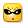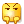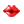LOLTMD多功能助手 v9.07 免费版

软件介绍

LOLTMD多功能辅助是一款免费的lol多功能助手软件，这款软件提供了超多实用的辅助功能，包括补刀提示、技能cd计时、对方回城提示、惩戒提示、攻击范围显示、技能范围显示等。如果你上分总遇到坑队友，可以来西西下载这款软件试试一神带4坑，超神的感觉等着你。

[暗裔剑魔]

1=仲裁圣骑

2=霸天剑魔

3=狂鲨

[九尾妖狐]

1=高丽风情

2=暗影狐妖

3=焰尾妖狐

4=偶像歌手

5=勇者

6=风纪委员

7=电玩女神

8=炫彩皮肤1

9=炫彩皮肤2

10=炫彩皮肤3

11=炫彩皮肤4

12=炫彩皮肤5

13=炫彩皮肤6

14=星之守护者 阿狸

[暗影之拳]

1=蜂刺

2=绯红

3=足球宝贝

4=实习护士

5=鬼武姬

6=银色之牙

7=铁血女忍

8=刺身之拳

[牛头酋长]

1=暗黑灵魂

2=金牛座

3=斗牛

4=荒野镖客

5=巨角幻兽

6=地狱火

7=后场堡垒

8=黑暗骑士

9=SKT T1

10=哞利斯塔

11=炫彩皮肤.哞利斯塔1

12=炫彩皮肤.哞利斯塔2

13=炫彩皮肤.哞利斯塔3

14=炫彩皮肤.哞利斯塔4

15=炫彩皮肤.哞利斯塔5

16=炫彩皮肤.哞利斯塔6

17=炫彩皮肤.哞利斯塔7

18=炫彩皮肤.哞利斯塔8

[殇之木乃伊]

1=法老

2=狂欢

3=情绪摇滚

4=主人不要我了

5=王子不是我

6=小小骑士

7=殇之机器人

8=惊悚派对

[冰晶凤凰]

1=双重冰晶

2=联盟之喙

3=重甲猎鹰

4=钢铁之翼

5=黯晶凤凰

6=翼龙

7=庆典女皇

[黑暗之女]

1=哥特萝莉

2=小红帽

3=安妮梦游仙境

4=舞会公主

5=冰霜烈焰

6=安伯斯与提妮

7=科学怪熊的新娘

8=你看见过我的熊猫吗

9=甜心宝贝

10=海克斯科技

[寒冰射手]

1=极地女神

2=丛林侠盗

3=勇敢的心

4=女皇

5=紫晶射手

6=觅心女王

7=黑暗骑士

8=源计划 艾希

[铸星龙王]

1=灰烬领主

[沙漠皇帝]

1=星际之门

2=幽冥领主

3=SKT T1

4=司马懿 仲达

[星界游神]

1=长者之森

2=星界游神 巴德 恒星之耀

3=星界游神 巴德 流星之煌

4=星界游神 巴德 彗星之辉

5=冰雪游神

6=巴德・吟游诗人

发表评论查看所有评论(0)

昵称:
表情:字数: 0/500 (您的评论需要经过审核才能显示)

TOP
软件下载